# Uniformity statistics based on EMVA-1288

### Introduction

The European Machine Vision 1288 standard (EMVA 1288) is designed to test several key qualities of linear image sensors, including linearity, sensitivity (quantum efficiency), noise, dark current, nonuniformities, and spectral sensitivity. Imatest 2020.2 performs a subset of these measurements, including temporal noise and spatial nonuniformities in flat-field images. Results including Photo Response Nonuniformity and Dark Signal Nonuniformity (PRNU or DSNU for light and dark (“lens cap on”) images, respectively), can be calculated from batches of flat-field images in the Uniformity and Uniformity Interactive modules.

These measurements are derived from the EMVA 1288 standard and have similar numerical results, but they are not strictly EMVA 1288-compliant because the methodology is different. A sensor Dynamic Range measurement, is also designed to give results comparable to EMVA 1288, and again, it’s far from compliant.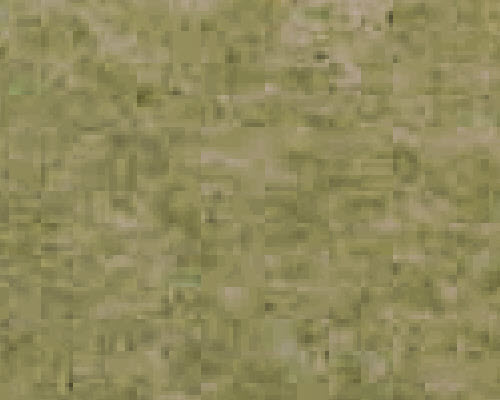Noise from a real image, shown with increased contrast; 4 display pixels per image pixel. Some 8×8 blocking from (low quality) JPEG processing  is visible.

Image sensor noise is a random variation of pixel levels caused by a number of factors— photons (shot noise), thermal (Johnson noise), dark current noise, and variations in the sensitivity and gain of pixels in the image sensor, sometimes called fixed pattern noise (which we will call spatial nonuniformity here. “Nonuniformity” is preferred in the EMVA standard (§4).

Image sensor noise can be decomposed into two fundamental factors.

• Temporal noise, which is random noise that varies from image to image.
• Spatial noise or nonuniformity,  caused by nonuniform pixel sensitivity and gain, which is consistent from image to image.

There are two ways of measuring temporal noise. Method 1 subtracts two images acquired under identical conditions, then divides by √2. Method 2 requires a large number of images. This method is used by the EMVA calculation because multiple images are also required to obtain spatial nonuniformity.

Calculating temporal noise and nonuniformity requires that multiple images be captured. (L ≥ 16 is the minimum recommended in EMVA §8; 100-400 is better, but the Imatest calculation will work with as few as 4 images (good for testing-only). The standard recommends capturing a set of light images whose mean pixel level (in linear files with no gamma applied) is 50% of saturation (designated by subscript 50 in the document; we prefer “light”) and also a set of dark pixels (“lens cap on”; designated by subscript dark).

A note on EMVA 1288 equations: Imatest does not currently use photometric units, such as K = Digital Number (DN) per electron; η = quantum efficiency; μp = mean number of photons per pixel; μe = mean number of electrons per pixel ( μe = η μ). Imatest results are derived only from electrical measurements.

The notation in §8 is confusing, sometimes inconsistent, and often difficult to follow. For example, EMVA equation (42),  $$\langle y\rangle = \frac{1}{L}\sum_{l=0}^{L-1}y[l]$$ is not used after it is defined. The mean values μy.50 and μy.dark used in Eq. (46) are apparently derived from <y>.

Since there are many inconsistencies in the equations, and photometric units are often mixed with electrical or digital units, we are unable to follow the equations the standard exactly. The equations below represent actual Imatest calculations, which are intended to be equivalent to the standard measurements. We will try to follow some EMVA 1288 notation. μ represents the mean; s represents spatial standard deviations; σ represents temporal standard deviations.

Summary of the equations below:  For a set of L captured images y[l], where y represents each pixel in the image, calculate the sum and sum of squares of the images (or more precisely, each pixel in the image).

The mean of each individual pixel in L captured images is

$$\displaystyle\mu_s = \frac{1}{L}\sum_{l=0}^{L-1}y[l]$$

Spatial nonuniformity s is the standard deviation of μs.

The temporal noise variance (power) each individual pixel in the image is

$$\displaystyle\sigma_s^2 = \frac{1}{L-1}\sum_{l=0}^{L-1}\left(y[l] – \frac{1}{L}\sum_{l=0}^{L-1}y[l]\right)^2 = \frac{1}{L-1}\sum_{l=0}^{L-1}\left(y[l] – \mu_s \right)^2$$     EMVA (44)

This equation could be slow and tedious to evaluate, but there is a shortcut, described in the Wikipedia Variance page. In Wikipedia’s notation, $$E(x) = E[(x-E[x])^2] = E[x^2]-E[x]^2$$. In the EMVA notation,

$$\displaystyle\sigma_s^2 = \frac{1}{L}\sum_{l=0}^{L-1}y^2[l]\ – \left(\frac{1}{L}\sum_{l=0}^{L-1}y[l] \right)^2$$

What this means in practice is that if you select L files, each file is read, then the pixel values are added to an array that contains the sum of pixel values, and the square of pixel values are added to an array that contains the sum of squares of pixel values. Individual images are not processed further. This process is fast and efficient.

 Observation: For an arbitrary image (not just flat field images specified by EMVA 1288), μs is the familiar signal-averaged mean signal image, whose SNR is improved by 3*log2(L) dB for L samples. Noise voltage σs also forms an image (the noise image), which is much less familiar than the signal image, but which can provide insight into image processing, for example for bilateral filtering, where sharpening/noise reduction, hence noise, varies over the image surface. Noise images are discussed in more detail in Measuring Temporal Noise.

The full EMVA 1288 Photo Response Nonuniformity (PRNU1288)  measurement uses a set of light images, designated by the subscript 50 or light, and a set of dark images, designated by the subscript dark. When a set of images is run, results are temporarily stored in imatest-v2.ini. PRNU1288 is only calculated when both light and dark are available.

$$\displaystyle PRNU_{1288} = \frac{\sqrt{\langle s_{y.light}\rangle ^2 – \langle s_{y.dark}\rangle ^2 } } { \langle \mu_{y.light}\rangle – \langle \mu_{y.dark}\rangle}$$

where <…> denotes the mean taken over the image.

Using only the light set of images, we can define PRNUlight, which is very close to PRNU1288.

$$\displaystyle PRNU_{light} = \langle s_{y.light}\rangle / \langle \mu_{y.light}\rangle$$

Dark Signal Nonuniformity is $$DSNU = \langle s_{y.dark}\rangle$$

Temporal noise (reported as a single number: mean for light or dark images) is $$\sigma_y = \langle \sigma_s\rangle$$.

### Method

Photograph a flat field, which is typically a highly even light source, such as a non-flickering LED lightbox. For the bright images, if exposure control is available, choose an exposure to linear images are about 50% of the maximum level (this would be about 73% of maximum for a gamma = 2.2 color space).

For saved image files, open Uniformity or Uniformity Interactive, and select a group of L (light or dark) files. A confirmation window will open. Be sure to select Read n files for pixel-based temporal noise (N >= 4). For EMVA-1288.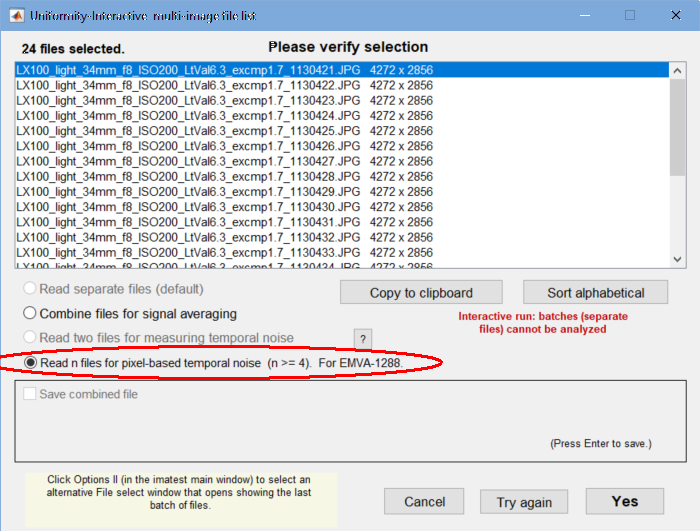File confirmation window. Be sure to select Read n files … for EMVA-1288.

A region selection window will open. Most of the time you’ll want to select the entire image (or use the same selection as the previous image, if you analyzed an image of the same size). Once you’ve made the selection, a progress bar will appear: reading multiple images goes surprisingly quickly.

For direct data acquisition, select the acquisition device with the Device Manager, which can be opened from the Settings dropdown menu in the Imatest main window, the Data tab in the box on the right, or the Uniformity Interactive Settings window. When you’ve made the selection, press  Save .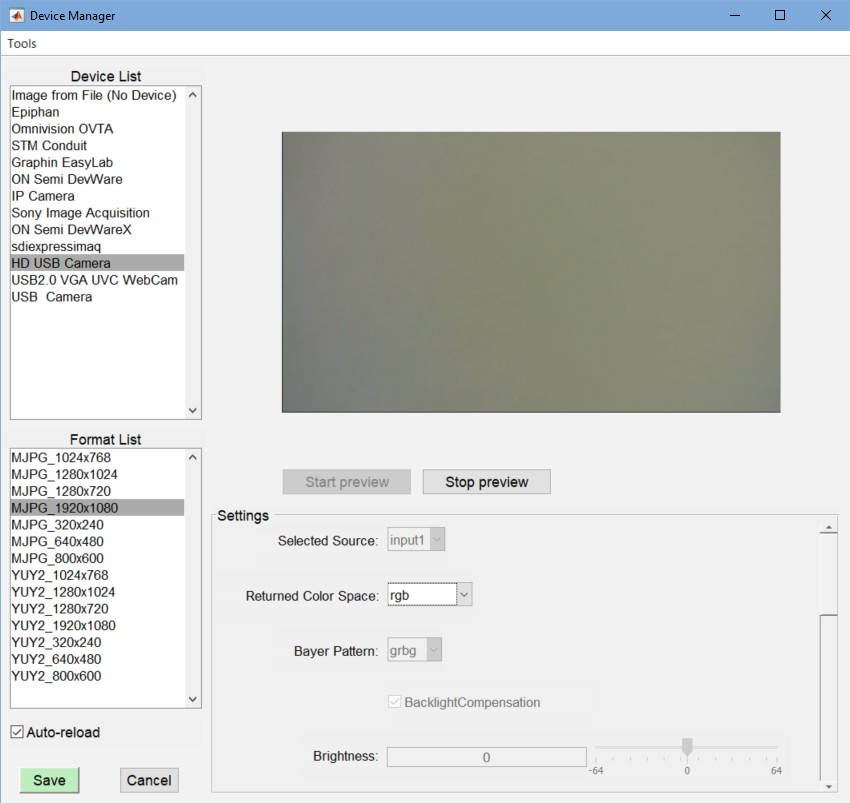Device Manager. HD USB camera (1920×1080 pixels) has been selected. Press  Save  when ready.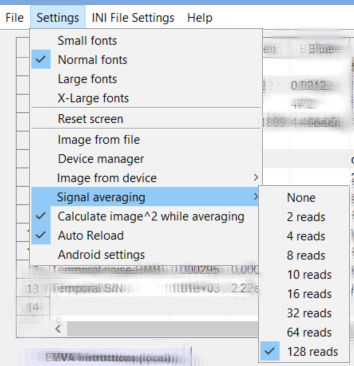If possible, prior to acquiring the data, in the Settings dropdown menu, Signal averaging should be set to the desired number, and Calculate image^2 while averaging (which has the same function as Read n files … for EMVA-1288, above) should be checked. (This name may change.) It should be easy to repeat the data acquisition using the Acquire image (Read Image File) button on the bottom-left of the Uniformity Interactive window.

In the More settings window, EMVA 1288 should be set to EMVA 1288 calculations: Box filter. The 5×5 Box filter is a highpass filter, described in §C.5, designed to suppress low-frequency spatial variations.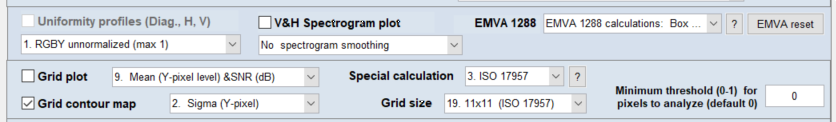The  EMVA reset  button on the right deletes EMVA 1288 batch results stored in imatest-v2.ini.

 Photograph a light or dark flat field image. For direct data acquisition, use the Device manager to select the device. Open Uniformity Interactive. In the Settings dropdown menu, select Signal averaging, and choose the number of images to average (must be ≥; 128 preferred), and be sure Calculate image^2 while averaging is checked. Then acquire the image. From saved image files, select a batch of files, then be sure to select Read n files for pixel-based temporal noise (N >= 4). For EMVA-1288 in the confirmation window. In the Uniformity or Uniformity Interactive More settings window, select EMVA 1288 calculations, Box filter. For Uniformity, select the plots.

### Results

Several plots, selected in the Display dropdown window, contain EMVA 1288 results. These include Histograms, Accum. Histograms, V&H Spectrum, EMVA & Histogram, and Temporal noise image

EMVA & Histogram  contains a summary of results.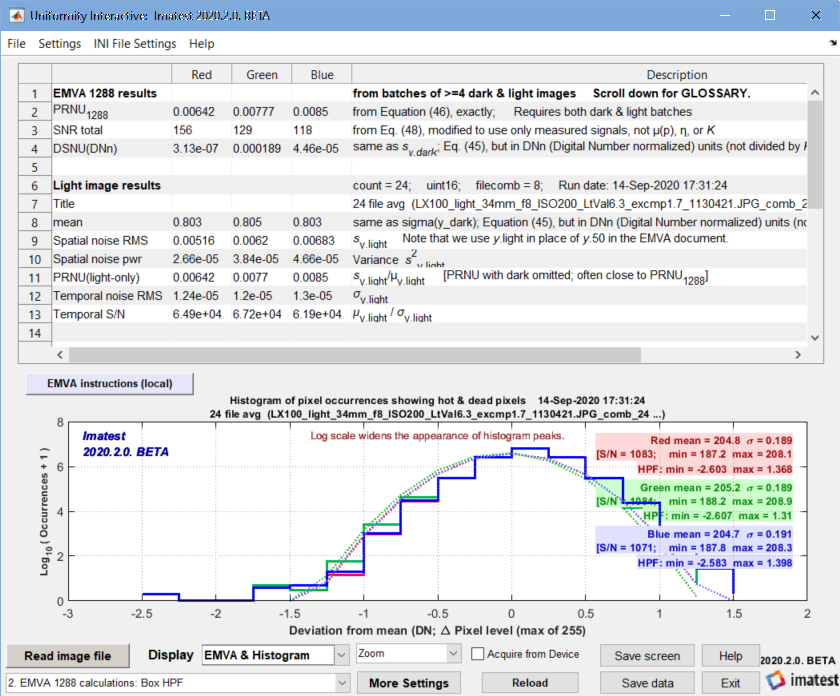EMVA & Histogram results. (Some results are visible when the window is scrolled down.)

When this plot is selected in Uniformity (the fixed module), the histogram (available in other plots) is omitted so the entire output can be seen.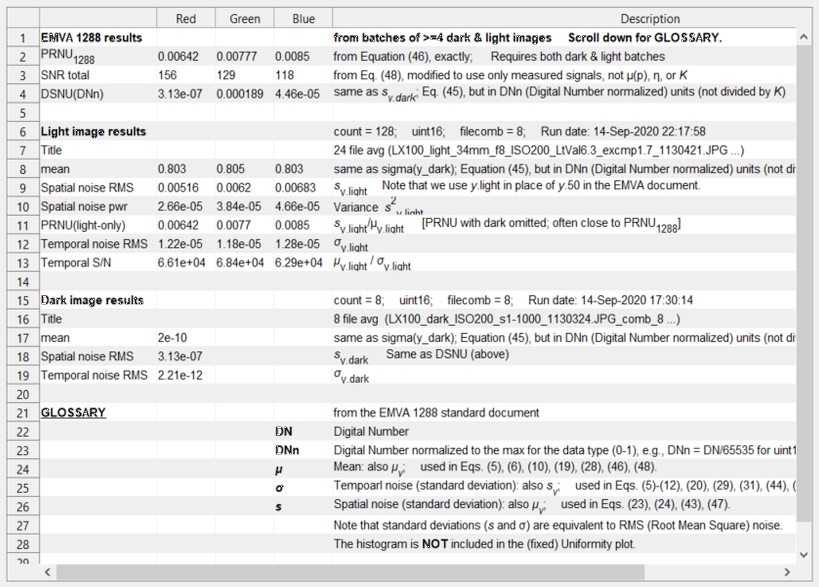EMVA results from Uniformity (not Interactive)

Some additional results are of interest.

Standard histograms — illustrated in EMVA §8.4, Figure 13. Can be calculated for light or dark images. Here are two examples, without and with the box filter, which is recommended by EMVA 1288 (§C.5) for removing low spatial frequency nonuniformities.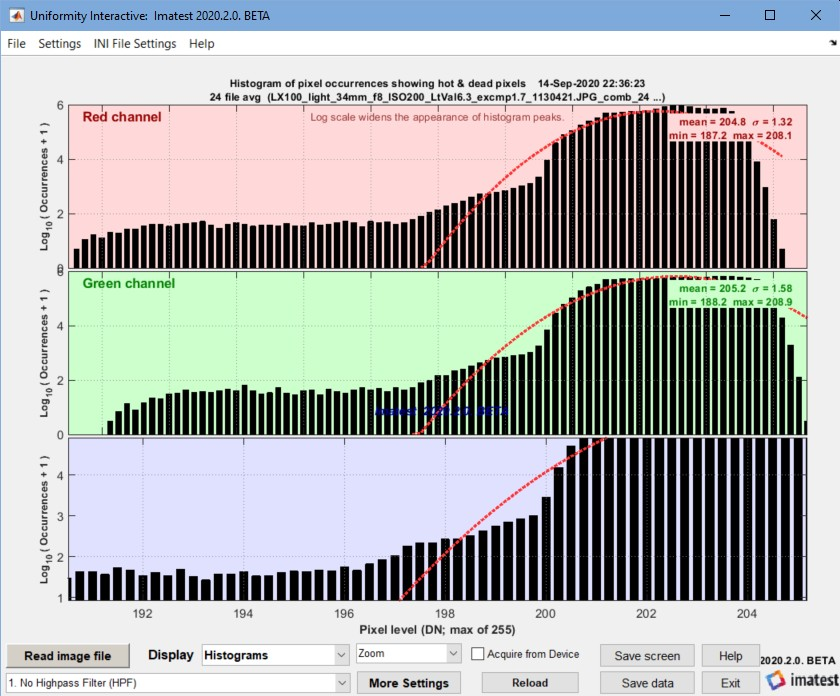Histogram without Box (lowpass) filter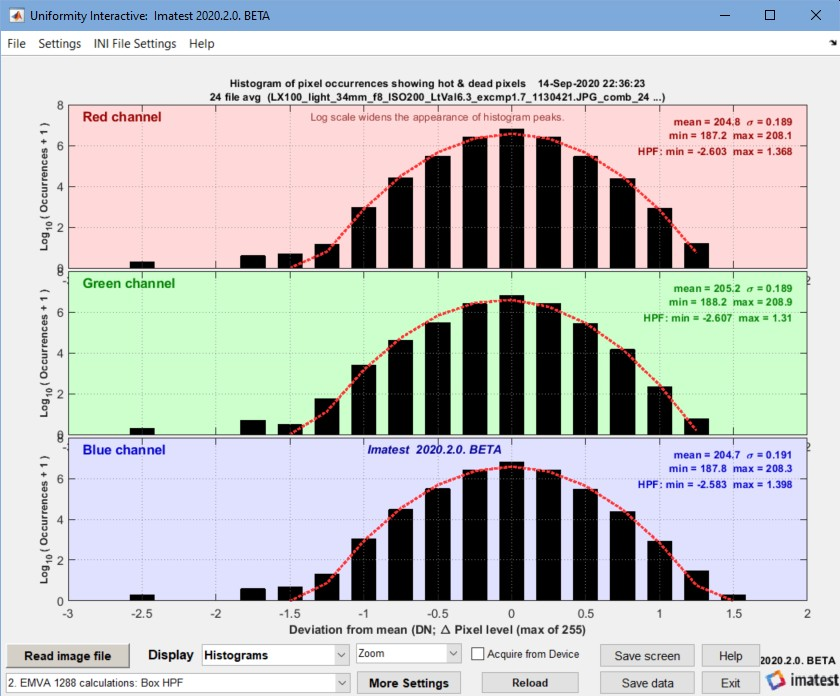Histogram with Box (lowpass) filter

Accumulated histograms — illustrated in EMVA §8.4, Figure 14. We are not sure how to use them. Does not contain EMVA required results.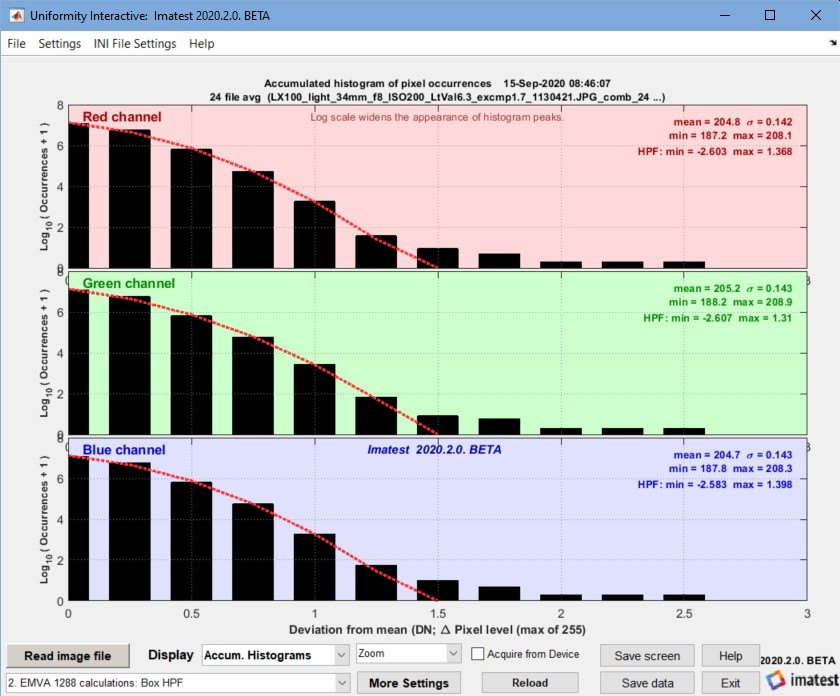Accumulated Histogram (with box filter)

Horizontal and vertical spectrograms and profiles —  described in EMVA §8.3 and 8.4. Combined here. These plots do not contain EMVA required results. Several smoothing settings are available.

Temporal noise image — Temporal noise power (variance) σ2 and RMS voltage σ as defined in the equations in the green box (above) are calculated for each pixel, i.e., they are images. For the flat-field images used in the EMVA calculations, temporal noise images are not of much interest, but they are of great interest for test chart images because they show how image processing varies across the image field. (When bilateral filtering is applied, noise is typically larger near edges than in smooth areas.) See Temporal noise for more details.